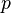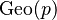# Geometric distribution facts for kids

Kids Encyclopedia Facts

In probability, the geometric distribution with probability of success$p$, written$\operatorname{Geo}(p)$, is a discrete probability distribution defined on non-negative integers. It is used to model the number of trials needed to obtain a first success, where the probability of success of each trial is$p$.

An example involving geometric distribution is the number of times a die needs to be tossed in order to get a first heads.

The geometric distribution is the discrete analogue of the exponential distribution.

## Related pagesGeometric distribution Facts for Kids. Kiddle Encyclopedia.